### Section 4.4.7.1Teaching An Old Spline New Tricks

If we followed the section on splines covered under the lathe tutorial (see section "Understanding The Concept of Splines"), we know that there are two additional kinds of splines besides linear: the quadratic and the cubic spline. Sure enough, we can use these with prisms to make a more free form, smoothly curving type of prism.

There is just one catch, and we should read this section carefully to keep from tearing our hair out over mysterious too few points in prism messages which keep our prism from rendering. We can probably guess where this is heading: how to close a non-linear spline. Unlike the linear spline, which simply draws a line between the last and first points if we forget to make the last point equal to the first, quadratic and cubic splines are a little more fussy.

First of all, we remember that quadratic splines determine the equation of the curve which connects any two points based on those two points and the previous point, so the first point in any quadratic spline is just a control point and won't actually be part of the curve. What this means is: when we make our shape out of a quadratic spline, we must match the second point to the last, since the first point is not on the curve - it's just a control point needed for computational purposes.

Likewise, cubic splines need both the first and last points to be control points, therefore, to close a shape made with a cubic spline, we must match the second point to the second from last point. If we don't match the correct points on a quadratic or cubic shape, that's when we will get the too few points in prism error. POV-Ray is still waiting for us to close the shape, and when it runs out of points without seeing the closure, an error is issued.

Confused? Okay, how about an example? We replace the prism in our last bit of code with this one (see file prismdm2.pov).

prism { cubic_spline 0, // sweep the following shape from here ... 1, // ... up through here 6, // the number of points making up the shape ... < 3, -5>, // point#1 (control point... not on curve) < 3, 5>, // point#2 ... THIS POINT ... <-5, 0>, // point#3 < 3, -5>, // point#4 < 3, 5>, // point#5 ... MUST MATCH THIS POINT <-5, 0> // point#6 (control point... not on curve) pigment { Green } }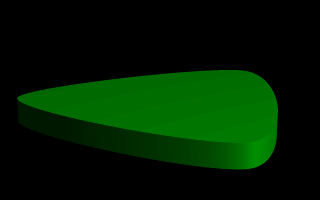A cubic, triangular prism shape.

This simple prism produces what looks like an extruded triangle with its corners sanded smoothly off. Points two, three and four are the corners of the triangle and point five closes the shape by returning to the location of point two. As for points one and six, they are our control points, and aren't part of the shape - they're just there to help compute what curves to use between the other points.

### Section 4.4.7.2Smooth Transitions

Now a handy thing to note is that we have made point one equal point four, and also point six equals point three. Yes, this is important. Although this prism would still be legally closed if the control points were not what we've made them, the curve transitions between points would not be as smooth. We change points one and six to <4,6> and <0,7> respectively and re-render to see how the back edge of the shape is altered (see file prismdm3.pov).

To put this more generally, if we want a smooth closure on a cubic spline, we make the first control point equal to the third from last point, and the last control point equal to the third point. On a quadratic spline, the trick is similar, but since only the first point is a control point, make that equal to the second from last point.

### Section 4.4.7.3Multiple Sub-Shapes

Just as with the polygon object (see section "Polygon Object") the prism is very flexible, and allows us to make one prism out of several sub-prisms. To do this, all we need to do is keep listing points after we have already closed the first shape. The second shape can be simply an add on going off in another direction from the first, but one of the more interesting features is that if any even number of sub-shapes overlap, that region where they overlap behaves as though it has been cut away from both sub-shapes. Let's look at another example. Once again, same basic code as before for camera, light and so forth, but we substitute this complex prism (see file prismdm4.pov).

prism { linear_sweep cubic_spline 0, // sweep the following shape from here ... 1, // ... up through here 18, // the number of points making up the shape ... <3,-5>, <3,5>, <-5,0>, <3, -5>, <3,5>, <-5,0>, // sub-shape #1 <2,-4>, <2,4>, <-4,0>, <2,-4>, <2,4>, <-4,0>, // sub-shape #2 <1,-3>, <1,3>, <-3,0>, <1, -3>, <1,3>, <-3,0> // sub-shape #3 pigment { Green } }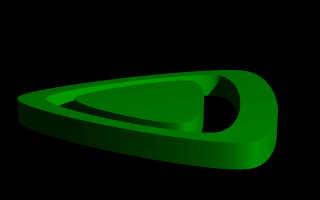Using sub-shapes to create a more complex shape.

For readability purposes, we have started a new line every time we moved on to a new sub-shape, but the ray-tracer of course tells where each shape ends based on whether the shape has been closed (as described earlier). We render this new prism, and look what we've got. It's the same familiar shape, but it now looks like a smaller version of the shape has been carved out of the center, then the carved piece was sanded down even smaller and set back in the hole.

Simply, the outer rim is where only sub-shape one exists, then the carved out part is where sub-shapes one and two overlap. In the extreme center, the object reappears because sub-shapes one, two, and three overlap, returning us to an odd number of overlapping pieces. Using this technique we could make any number of extremely complex prism shapes!

### Section 4.4.7.4Conic Sweeps And The Tapering Effect

In our original prism, the keyword linear_sweep is actually optional. This is the default sweep assumed for a prism if no type of sweep is specified. But there is another, extremely useful kind of sweep: the conic sweep. The basic idea is like the original prism, except that while we are sweeping the shape from the first height through the second height, we are constantly expanding it from a single point until, at the second height, the shape has expanded to the original points we made it from. To give a small idea of what such effects are good for, we replace our existing prism with this (see file prismdm5.pov):

prism { conic_sweep linear_spline 0, // height 1 1, // height 2 5, // the number of points making up the shape... <4,4>,<-4,4>,<-4,-4>,<4,-4>,<4,4> rotate <180, 0, 0> translate <0, 1, 0> scale <1, 4, 1> pigment { gradient y scale .2 } }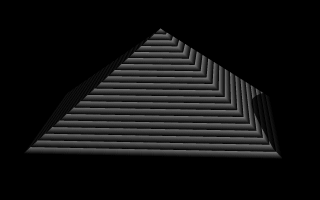Creating a pyramid using conic sweeping.

The gradient pigment was selected to give some definition to our object without having to fix the lights and the camera angle right at this moment, but when we render it, we what we've created? A horizontally striped pyramid! By now we can recognize the linear spline connecting the four points of a square, and the familiar final point which is there to close the spline.

Notice all the transformations in the object declaration. That's going to take a little explanation. The rotate and translate are easy. Normally, a conic sweep starts full sized at the top, and tapers to a point at y=0, but of course that would be upside down if we're making a pyramid. So we flip the shape around the x-axis to put it rightside up, then since we actually orbitted around the point, we translate back up to put it in the same position it was in when we started.

The scale is to put the proportions right for this example. The base is eight units by eight units, but the height (from y=1 to y=0) is only one unit, so we've stretched it out a little. At this point, we're probably thinking, "why not just sweep up from y=0 to y=4 and avoid this whole scaling thing?"

That is a very important gotcha! with conic sweeps. To see what's wrong with that, let's try and put it into practice (see file prismdm6.pov). We must make sure to remove the scale statement, and then replace the line which reads

1, // height 2 with

1, // height 2

This sets the second height at y=4, so let's re-render and see if the effect is the same.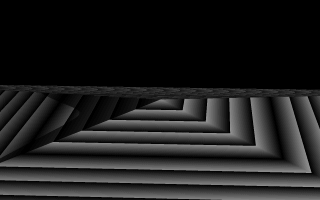Choosing a second height larger than one for the conic sweep.

Whoa! Our height is correct, but our pyramid's base is now huge! What went wrong here? Simple. The base, as we described it with the points we used actually occurs at y=1 no matter what we set the second height for. But if we do set the second height higher than one, once the sweep passes y=1, it keeps expanding outward along the same lines as it followed to our original base, making the actual base bigger and bigger as it goes.

To avoid losing control of a conic sweep prism, it is usually best to let the second height stay at y=1, and use a scale statement to adjust the height from its unit size. This way we can always be sure the base's corners remain where we think they are.

That leads to one more interesting thing about conic sweeps. What if we for some reason don't want them to taper all the way to a point? What if instead of a complete pyramid, we want more of a ziggurat step? Easily done. After putting the second height back to one, and replacing our scale statment, we change the line which reads

0, // height 1 to

0, // height 1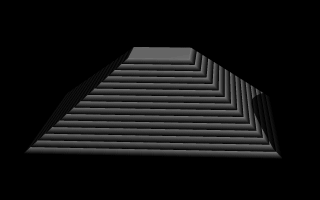Increasing the first height for the conic sweep.

When we re-render, we see that the sweep stops short of going all the way to its point, giving us a pyramid without a cap. Exactly how much of the cap is cut off depends on how close the first height is to the second height.

Next Section